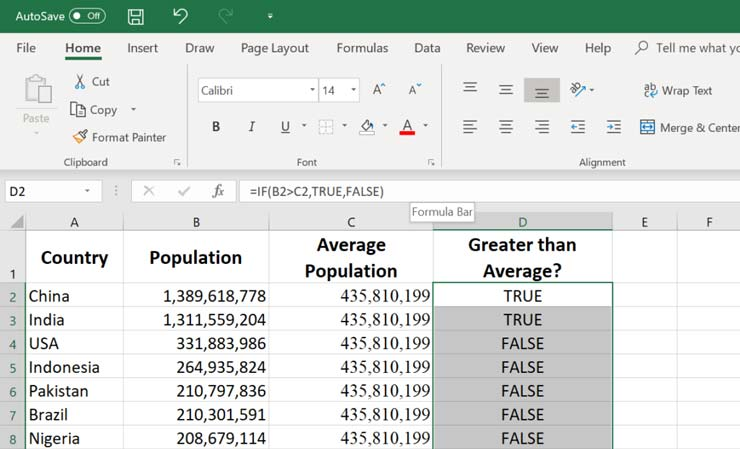# You probably won’t be excited about learning formulas. Most of us have a laid back attitude when it comes to painting a picture about ourselves. That’s why we use Microsoft Excel to do the math.

Do not look at these Excel formulas through the eyes of mathematics. These formulas can save you from doing manual calculations. After reading this educational article, you will acquire the following skills:

• Calculate the average score of your exams.
• Quickly calculate the invoices you have issued to your customers.
• Use basic statistics to review a set of data for trends and indicators.

## Formula Basics

Before we get started, let’s look at how to use formulas in Microsoft Excel. The following tips will help you master Excel (even if you do not deal with mathematical formulas in Excel).

To type a formula, click a cell in Microsoft Excel and type an equal sign.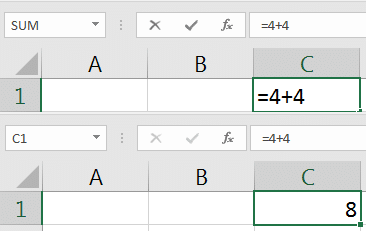After the equal sign, you can put different things inside the cell. Type 4 + 4 = as your first formula and press enter to see the result. Excel will display the number 8 and the formula will remain on the screen.

### 2- Formulas are shown in the Excel formula bar

When you type a formula in a cell, you can see the result by pressing the Enter key. But by selecting a cell, you can see the formula of that cell in the formula bar.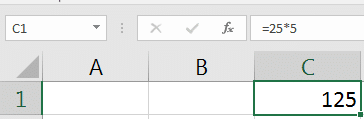To display the formula, just click on a cell in Excel, such as the multiplication formula in the example above, which results in 125.

In the example above, the number “8” will be displayed, but when we click on it, the formula bar shows that it adds cell 4 to 4.

### 3- How to make a formula

In the example above, we typed a simple addition formula to add two numbers. However, you do not always have to type numbers, you can refer to other cells.

Excel is a network of cells consisting of columns from left to right, each dedicated to a letter, as well as numbered rows. Each cell is the intersection of a row and a column. For example, the cell that intersects column A and row 3 is called A3.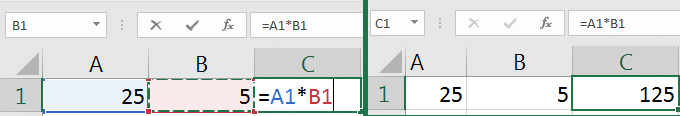Excel formulas can be written based on values ​​in several cells, such as multiplying A1 and B1 to calculate the value of C1, which is equal to 125.

Suppose I have two cells with prime numbers like 1 and 2 that are in cells A2 and A3. When I type a formula, I can start with “=” as usual. Then continue it as follows:

= A2 + A3

… To add those two numbers together. It is very common to have a sheet containing quantities, and a separate sheet for calculations. Keep this in mind when working with this article. For each of the formulas, you can reference cells, or type numeric values ​​directly into the formula.

If you want to change the formula you typed before, double-click the cell and reset the values.

## Account in Excel

In this section, we will examine Excel mathematical formulas and how to use them. Let’s start by examining basic arithmetic formulas. These formulas are the basis of mathematical operations.

Addition and subtraction are two basic mathematical formulas in Excel. If you want to calculate your monthly business cost list or digitally balance your ledger, you need to use addition and subtraction.

For practice, you can use the “Add and Subtract” tab in the workbook.

Example:

48 + 24 =

Or the reference values ​​of the following cells:

= A2 + A3

Tip: Try adding five or six values ​​to see what happens. Separate each item with a plus sign.To add values ​​in Excel, type two numbers and the “+” sign or add two reference cells together.

Subtract the values

Example:

75-25 +10 =

Or the reference values ​​of the following cells:

= A3-A2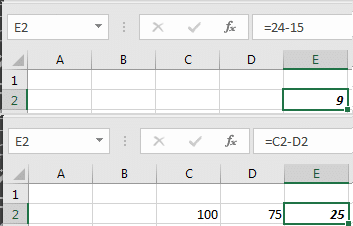Subtract the two values ​​in Excel by typing the numbers directly in the box, or by separating them with a “-” sign or referencing two cells with a “-” sign between them.

### ۲- ضرب

Use * to multiply values. Use multiplication instead of adding values ​​many times.

You can use the “Multiply” tab in the workbook to practice.

Example:

5 * 4 =

Or the reference values ​​of the following cells:

= A2 * A3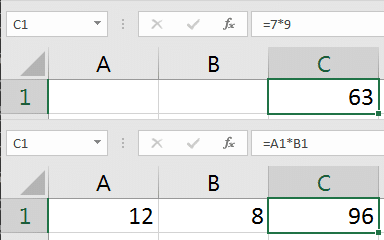Multiplication in Excel uses the * symbol between two values ​​or a reference cell.

### 3- Division

Dividing is useful in separating groups from each other. Use “/” to divide the numbers or values ​​in the cells on your page.

You can use the “Devide” tab in the workbook to practice.

Example:

20/10 =

Or reference values ​​in cells with:

= A5 / B2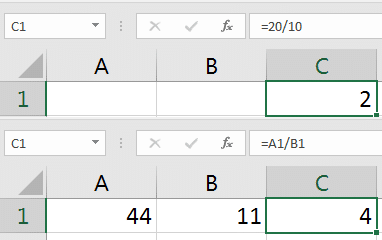Divide the values ​​in Excel with /, with the values ​​in the formula or between two cells.

## Basic statistics

You can use the “Basic Statistics” tab in your workbook to practice.

Now that you know the basics of math, let’s move on to more advanced ones. Basic statistics are useful for reviewing a set of data and drawing conclusions about it. In the next section, we will review some simple and common statistical formulas.

### 1- Average

To use the mean formula in Excel, your formula with

AVERAGE (=

Start and then enter the values. Separate numbers with commas. When you enter Enter, Excel will calculate the average and display the output.

= AVERAGE (1,3,5,7,10)

The best way to calculate the mean is to enter your values ​​in separate cells in a single column.

= AVERAGE (A2: A5)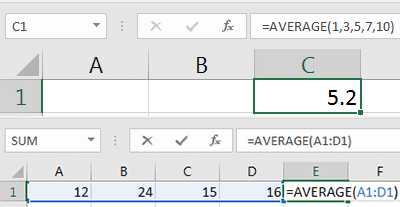Use the formula AVERAGE = to calculate the average of a set of comma separated values ​​or a set of cells as in the example above.

### 2- Middle

The middle of a set of data is the value in the middle of it. If you sort the numeric values ​​from small to large, the middle will be in the middle of that list.

= MEDIAN (1,3,5,7,10)

I recommend typing your values ​​into a set of cells, and then using the middle formula above the cells that contain the typed values.

= MEDIAN (A2: A5)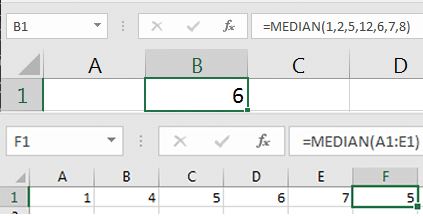Use the MEDIAN = formula to find the middle, or the formula in the list of cells that have values.

### 3- Minimum (minimum)

Use the MIN formula in Excel to find the minimum set of data. To find the smallest value in a data set, you can use the MIN formula with a set of numbers, separated by commas. This method will be very useful when working with large data sets.

= MIN (1,3,5,7,9)

If you want to find the minimum set of data, you can use the following formula:

= MIN (A1: E1)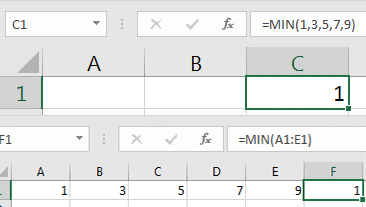Use the Excel MIN formula to find the minimum set of values ​​separated by commas or a large number of cells.